﻿ 威布尔杂波下扩展目标检测

# 威布尔杂波下扩展目标检测Extended Target Detection under Weibull Clutter

Abstract: Target detection under sea clutter is widely used in military and civilian applications and has broad prospects. As the resolution of the radar increases, the sea clutter exhibits a non-Gaussian amplitude, which brings new challenges to the detection of radar targets. At present, in the research of wide-band radar target detection, the commonly used broadband radar sea clutter amplitude distribution models are: lognormal distribution, Weibull distribution, K distribution and so on. This paper mainly analyzes the detection problem of wide-band targets in the sea clutter background of Weibull distribution, and proposes a new detection algorithm for extended targets under Weibull distribution: firstly, two parameters of Weibull distribution are estimated by moment estimation method. Then a new detection quantity is constructed combined with the amplitude accumulation detector to detect the target. It is found that the Weibull distribution can be converted into a standard exponential distribution by constructing a new detection quantity, and the problem is converted into an exponentially distributed broadband target in the background of the sea clutter. Through detecting problems and greatly simplifying the original problems, finally, the paper proves the effectiveness of the algorithm.

1. 引言

2. 基于矩估计的参数估计

${\mu }_{m}=E\left({X}^{l}\right)={\int }_{-\infty }^{+\infty }{x}^{m}f\left(x;{\theta }_{1},{\theta }_{2},\cdots ,{\theta }_{k}\right)\text{d}x$ ，X连续 (1)

${\mu }_{m}=E\left({X}^{l}\right)=\underset{x\in {R}_{x}}{\sum }{x}^{m}p\left(x;{\theta }_{1},{\theta }_{2},\cdots ,{\theta }_{k}\right)\text{d}x$ ，X离散(2)

${A}_{m}=\frac{1}{n}\underset{i=1}{\overset{n}{\sum }}{X}_{i}^{m}$ (3)

$\left\{\begin{array}{l}{\mu }_{1}={\mu }_{1}\left({\theta }_{1},{\theta }_{2},\cdots ,{\theta }_{k}\right),\\ {\mu }_{2}={\mu }_{2}\left({\theta }_{1},{\theta }_{2},\cdots ,{\theta }_{k}\right),\\ \text{\hspace{0.17em}}\text{\hspace{0.17em}}\text{\hspace{0.17em}}\text{\hspace{0.17em}}\text{\hspace{0.17em}}\text{\hspace{0.17em}}\text{\hspace{0.17em}}\text{\hspace{0.17em}}\text{\hspace{0.17em}}\text{\hspace{0.17em}}\text{\hspace{0.17em}}\text{\hspace{0.17em}}\text{\hspace{0.17em}}\text{\hspace{0.17em}}⋮\\ {\mu }_{k}={\mu }_{k}\left({\theta }_{1},{\theta }_{2},\cdots ,{\theta }_{k}\right).\end{array}$ (4)

$A\left({X}^{m}\right)=\frac{1}{n}\underset{i=1}{\overset{n}{\sum }}{X}_{i}^{m}$ (5)

$E\left({X}^{m}\right)=A\left({X}^{m}\right)$ (6)

$f\left(x\right)=\frac{\beta }{\eta }{\left(\frac{x}{\eta }\right)}^{\beta -1}\mathrm{exp}\left(-{\left(\frac{x}{\eta }\right)}^{\beta }\right)$ (7)

$F\left(x\right)=1-\mathrm{exp}\left(-{\left(\frac{x}{\eta }\right)}^{\beta }\right)$ (8)

$E\left({X}^{m}\right)=\underset{0}{\overset{\infty }{\int }}{x}^{m}f\left(x\right)\text{d}x=\underset{0}{\overset{\infty }{\int }}{x}^{m}\frac{\beta }{\eta }{\left(\frac{x}{\eta }\right)}^{\beta -1}\mathrm{exp}\left(-{\left(\frac{x}{\eta }\right)}^{\beta }\right)\text{d}x={\eta }^{m}\Gamma \left(\frac{m}{\beta }+1\right)$ (9)

$\left\{\begin{array}{l}\eta \Gamma \left(\frac{1}{\beta }+1\right)=E\left(X\right)\\ {\eta }^{2}\Gamma \left(\frac{2}{\beta }+1\right)=E\left({X}^{2}\right)\end{array}$ (10)

$\left\{\begin{array}{l}\beta =\frac{\pi E\left({X}^{2}\right)E\left({X}^{-2}\right)/E\left(X\right)E\left({X}^{-1}\right)}{\sqrt{{\left(E\left({X}^{2}\right)E\left({X}^{-2}\right)\right)}^{2}-{\left(E\left(X\right)E\left({X}^{-1}\right)\right)}^{2}}}\\ \eta =\frac{E\left(X\right)}{\Gamma \left(1+\frac{1}{\beta }\right)}\end{array}$ (11)

3. 威布尔分布的非相干积累

${X}_{1},{X}_{2},\cdots ,{X}_{N}$ 为N个杂波样本且独立同分布，经非相干积累后随机变量 $\underset{i=1}{\overset{N}{\sum }}{X}_{i}$ 的PDF为 ${p}_{N}\left(x\right)$${X}_{i}\left(i=1,2,\cdots ,N\right)$ 的矩母函数为

$M\left(s\right)=E\left({\text{e}}^{s{X}_{i}}\right)$ (12)

$M\left(s\right)={\int }_{0}^{+\infty }{\text{e}}^{sx}\frac{c}{\eta }{\left(\frac{x}{\eta }\right)}^{\beta -1}{\text{e}}^{-{\left(\frac{x}{\eta }\right)}^{c}}\text{d}x$ (13)

$M\left(s\right)=\underset{n=0}{\overset{+\infty }{\sum }}\frac{{t}^{n}}{n!}E\left[{X}^{n}\right]$(14)

$M\left(s\right)=\underset{n=0}{\overset{+\infty }{\sum }}\frac{{s}^{n}}{n!}{\eta }^{n}\Gamma \left(\frac{n}{\beta }+1\right)$ (15)

$\underset{i=1}{\overset{N}{\sum }}{X}_{i}$ 的矩母函数为

 (16)

4. 算法设计

4.1. 问题描述

$f\left({s}_{n}\right)=\frac{{s}_{n}^{\left(m-1\right)}{\text{e}}^{-{s}_{n}/2}}{{2}^{m}\Gamma \left(m\right)}$ , ${s}_{n}>0$ (17)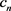是威布尔分布，表示背景杂波，这里采用两步法，先假设杂波参数 $\eta ,\beta$ 已知，再通过背景杂波进行估计得到。设目标回波的距离像分布不超过L个距离单元，对检测窗内的数据建立如下检测模型：

$\left\{\begin{array}{l}{H}_{0}:{z}_{n}={c}_{n};\\ {H}_{1}:{z}_{n}={s}_{n}+{c}_{n};\end{array}n=1,2,3,\cdots ,L$ (18)

4.2. 似然比检验

NP 准则下，假设检验式(16)的最优检测器为似然比检测器  (Likelihood Ratio Test, LRT)，其表达式为：

$\underset{n=1}{\overset{L}{\prod }}\frac{p\left({z}_{n}|{H}_{1}\right)}{p\left({z}_{n}|{H}_{0}\right)}\underset{{H}_{0}}{\overset{{H}_{1}}{\gtrless }}{T}_{h}$ (19)

$\underset{n=1}{\overset{L}{\sum }}f\left({z}_{n}\right)\underset{{H}_{0}}{\overset{{H}_{1}}{\gtrless }}\mathrm{ln}{T}_{h}$ (20)

${H}_{0}$ 假设下，有

$p\left({z}_{n}|{H}_{0}\right)=f\left({z}_{n}\right)=\frac{\beta }{\eta }{\left(\frac{{z}_{n}}{\eta }\right)}^{\beta -1}\mathrm{exp}\left(-{\left(\frac{{z}_{n}}{\eta }\right)}^{\beta }\right)$ (21)

${H}_{1}$ 假设下，有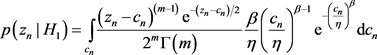(22)

4.3. 指数分布与 $Gamma\left(n,\lambda \right)$ 分布的关系

$n=1$ 时，

$Gamma\left(1,\lambda \right)=Exp\left(\lambda \right)$ (23)

${S}_{k+1}={S}_{k}+{X}_{k+1}$ ，其中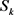服从 $Gamma\left(k,\lambda \right)$${X}_{k+1}$ 服从 $Exp\left(\lambda \right)$

$\mathrm{Pr}\left({S}_{k+1}=x\right)={\int }_{0}^{x}\mathrm{Pr}\left({S}_{k}=y\right){P}_{r}\left({X}_{k+1}=x-y\right)\text{d}y={\int }_{0}^{x}\frac{{\lambda }^{k}}{\Gamma \left(k\right)}{y}^{k-1}{\text{e}}^{-\lambda y}×\lambda {\text{e}}^{-\lambda \left(x-y\right)}\text{d}y=\frac{{\lambda }^{k+1}}{\Gamma \left(k+1\right)}{x}^{k}\text{e}$ (24)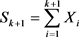服从 $Gamma\left(k+1,\lambda \right)$ 分布。

4.4. 检测器设计

$p\left({x}_{n}|{H}_{0}\right)=\frac{\beta }{\eta }{\left(\frac{{x}_{n}}{\eta }\right)}^{\beta -1}\mathrm{exp}\left(-{\left(\frac{{x}_{n}}{\eta }\right)}^{\beta }\right)$ (25)

${y}_{i}={\left(\frac{{x}_{i}}{\eta }\right)}^{\beta },\text{\hspace{0.17em}}i=1,2,\cdots ,L$ (26)

$F\left({y}_{i}\right)=1-\mathrm{exp}\left(-{y}_{i}\right)$ (27)

$f\left({y}_{i}\right)=\mathrm{exp}\left(-{y}_{i}\right)$ (28)

$t={y}_{1}+{y}_{2}+\cdots +{y}_{L}$ (29)

$\left\{\begin{array}{l}t\ge T,\text{\hspace{0.17em}}裁决目标存在\\ t

${P}_{\text{fa}}=\underset{T}{\overset{\infty }{\int }}f\left(t\right)\text{d}t$ (30)

1) 根据参考单元估计出威布尔分布的两个参数值；

2) 通过式(30)计算出门限值T；

3) 通过式(26)对检测单元进行变换；

4) 通过式(29)计算检测统计量t的值，将该值与门限值T进行比较得到结果。

5. 威布尔分布与指数分布仿真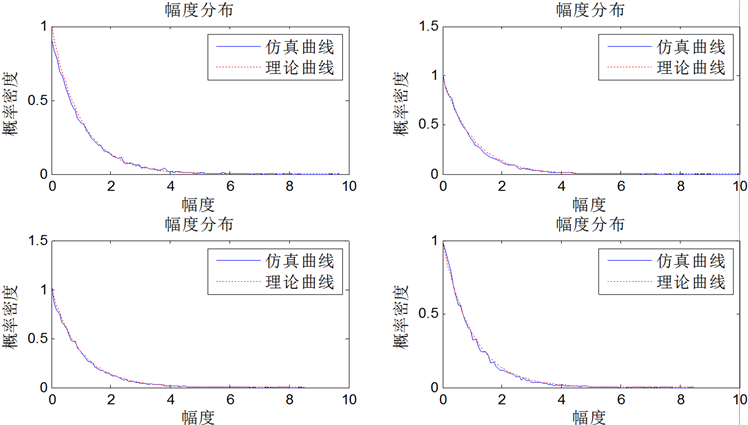Figure 1. PDF curve of standard exponential distribution and distribution after Weibull transformation

6. 算法仿真

6.1. 算法的稳定性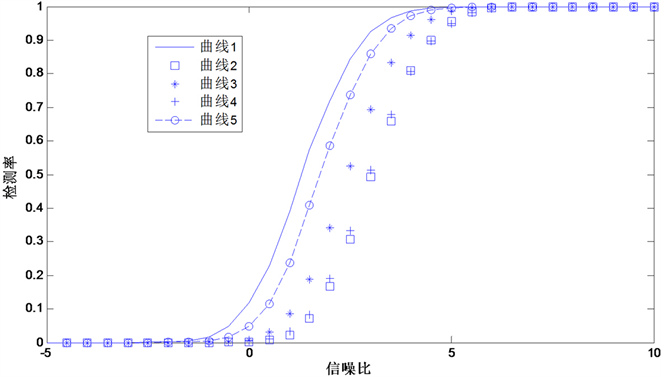Figure 2. Detection curve under different estimation conditions

6.2. 检测率仿真

6.3. 虚警率仿真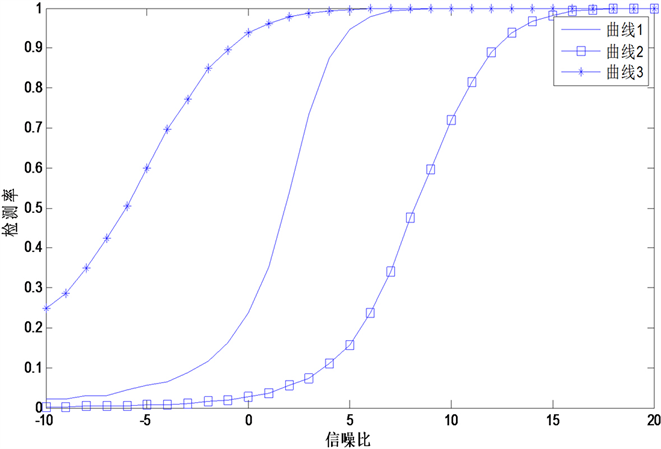Figure 3. Detection rate simulation curve

7. 结束语

 高铮, 张安清. 海杂波典型幅度分布模型的仿真分析[J]. 舰船电子工程, 2018, 38(9): 76-78+94.

 李丽, 王晓玲, 桂杰, 周哲海. 恒虚警技术处理方法综述[J]. 激光杂志, 2018, 39(1): 8-13.

 卫磊. 扩展目标的稳定检测技术研究[D]: [硕士学位论文]. 北京: 中国科学院大学(中国科学院光电技术研究所), 2017.

 陈远征, 周剑雄, 付强. 扩展目标的最优二进制检测[J]. 系统工程与电子技术, 2011, 33(1): 26-29.

 李建丽, 李海增, 邢美丽, 李江杰. 混合几何分布的矩估计[J]. 太原师范学院学报(自然科学版), 2017, 16(2): 21-23.

 王梓坤. 随机过程[M]. 西安: 西安交通大学出版社, 2006.

 张波. 高分辨海杂波背景下目标检测方法[[D]: [硕士学位论文]. 哈尔滨: 哈尔滨工程大学, 2016.

 雷刚, 邵可然, 李燕斌, 晏明. 威布尔杂波背景下的恒虚警检测[J]. 华中科技大学学报(自然科学版), 2008, 36(3): 129-132.

 申贝贝. 论特征函数的性质及应用[J]. 数学学习与研究, 2017(24): 7-8.

 问翔. 宽带雷达距离扩展目标检测算法研究[D]: [博士学位论文]. 西安: 西安电子科技大学, 2014.

 Besson, O. and Abramovich, Y. (2015) Sensitivity Analysis of Likelihood Ratio Test in K Distributed and/or Gaussian Noise. IEEE Signal Processing Letters, 22, 2329-2333.
https://doi.org/10.1109/LSP.2015.2480426

 何友等. 雷达目标检测与恒虚警处理[M]. 北京: 清华大学出版社, 2011.

Top